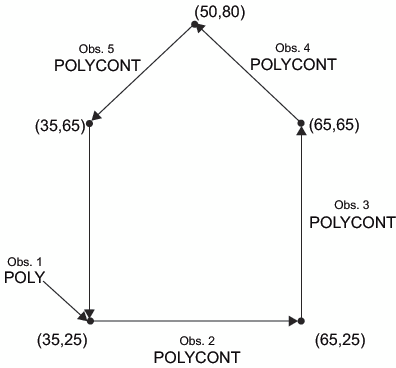# POLYCONT Function

Continues drawing a polygon begun with the POLY function. POLYCONT specifies each successive point in the polygon definition.

## Syntax

FUNCTION='POLYCONT';

### Associated Variables

COLOR='color'
specifies the polygon outline color. Color can be any SAS/GRAPH color name. You can specify an outline color only with the first POLYCONT command in the sequence; all subsequent POLYCONT commands ignore the COLOR variable. If you do not specify a color, the POLYCONT function uses the interior color that was specified with the POLY function.
WHEN='B' | 'A'
specifies when to draw the polygon in relation to other procedure output. See .
X=horizontal-coordinate
Y=vertical-coordinate
Z=depth-coordinate (PROC G3D only)
XC='character-type-horizontal-coordinate'
YC='character-type-vertical-coordinate'
specify a point on the outline of the polygon that is being created.
Restriction:Use the Z variable only with the G3D procedure.
XSYS='coordinate-system'
specifies the coordinate system for the X and XC variable. Use the XC variable only with XSYS='2'. See XSYS Variable for an explanation of coordinate-system.
YSYS='coordinate-system'
specifies the coordinate system for the Y and YC variable. Use the YC variable only with YSYS='2'. See YSYS Variable for an explanation of coordinate-system.
ZSYS='coordinate-system'
specifies the coordinate system for the Z variable. See ZSYS Variable for an explanation of coordinate-system.

## Details

The polygon definition is terminated by a new POLY command or by any of these functions:
 BAR LABEL PIEXY DRAW MOVE POINT DRAW2TXT PIE SYMBOL FRAME PIECNTR
Use POLY and POLYCONT together to draw a polygon. The (X, Y) observation from the POLY function and the last (X, Y) observation from POLYCONT are assumed to connect. Thus, you are not required to respecify the first point. For example, these statements draw a pentagon like the one in
```data house;
retain xsys ysys "3";
length function \$ 8;
/* start at the lower left corner */
function="poly"; x=35; y=25; output;
/* move to the lower right corner */
function="polycont"; x=65; y=25; output;
/* move to the upper right corner */
function="polycont"; x=65; y=65; output;
/* move to the center top*/
function="polycont"; x=50; y=80; output;
/* move to the upper left corner and complete the figure */
function="polycont"; x=35; y=65; output;
run;
proc ganno anno=house;
run;
quit;```
Pentagon Produced with the POLY and POLYCONT FunctionsMissing values for the X and Y variables that are specified with POLYCONT are interpreted differently from the way that they are interpreted with the other functions. Other functions use the missing values to request a default value. POLYCONT interprets a missing value as a discontinuity (that is, a hole) in the polygon. If you are not using the data coordinate system and you specify an X or Y value of –999 in a POLYCONT observation, the default of (XLAST, YLAST) is used. Missing values indicate holes and are handled identically in the Annotate facility and the GMAP procedure. See Displaying Map Areas and Response Data for more information about handling missing values.